# HSPT Quantitative : How to make non-geometric comparisons

## Example Questions

1 2 3 4 5 6 7 8 9 11 Next →

### Example Question #101 : Non Geometric Comparison

Examine (a), (b), and (c) to find the best answer:

a)percent ofb)c)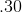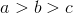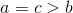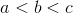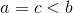Explanation:

To easily compare them, turn each expression into a decimal:

a)percent of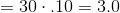b)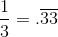c)Now it is clear that (a) is greater than (b), which is greater than (c)

### Example Question #102 : Non Geometric Comparison

Examine (a), (b), and (c) to find the best answer:

a)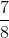b)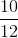c)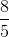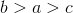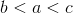Explanation:

Choose a common denominator to compare the fractions. One choice is, because its factors include, and:

a)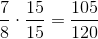b)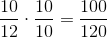c)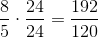It is now clear that (b) is smaller than (a), which is smaller than (c)

### Example Question #103 : Non Geometric Comparison

Examine (a), (b), and (c) to find the best answer:

a)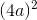b)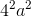c)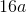*is a non-zero integer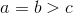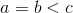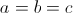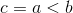Explanation:

a)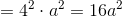b)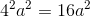c)Therefore (a) and (b) are equal. For all non-zero integers (whole numbers other than zero),will be smaller than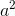, so (c) is smaller than (a) and (b).

### Example Question #104 : Non Geometric Comparison

Examine (a), (b), and (c) to find the best answer:

a)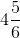b)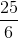c)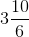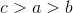Explanation:

Convert each into a proper fraction to compare the values:

a)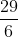b)c)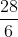1 2 3 4 5 6 7 8 9 11 Next →